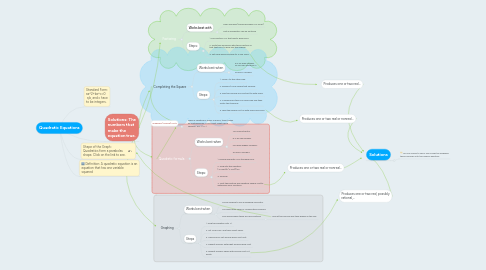Get Started. It's FreeQuadratic Equations## 6. Factoring

### 6.1. Works best with

6.1.1. small numbers (especially when a is small)

6.1.2. Not all quadratics can be factored

### 6.2. Steps:

6.2.1. 1. Find factors of c that add to give you b

6.2.2. 2. Write two binomials with those factors so that that FOIL to give you the original.

6.2.3. 3. Set each binomial equal to 0 and solve.

## 7. Completing the Square

### 7.1. Works best when

7.1.1. b is an even number so you can divide by 2

7.1.2. ALWAYS WORKS

### 7.2. Steps:

7.2.1. 1. Move c to the other side.

7.2.2. 2. Divide b/2 and square that number.

7.2.3. 3. Add the number you just got to both sides.

7.2.4. 4. Combine like terms on each side and then factor the trinomial.

7.2.5. 5. Take the square root of both sides and solve.

### 8.1. Works best when

8.1.1. You cannot factor

8.1.2. b is an odd number

8.1.3. you have bigger numbers

8.1.4. ALWAYS WORKS

### 8.2. Steps:

8.2.1. 1. Ensure quadratic is in standard form.

8.2.2. 2. Plug into the equation: (-b+sqrt(b^2-4ac))/2a

8.2.3. 3. Simplify

8.2.4. 4. Split the positive and negative square root to determine your solutions.

## 9. Graphing

### 9.1. Works best when

9.1.1. You're allowed to use a graphing calculator

9.1.2. You have really large or complicated numbers

9.1.3. Only works when there are real solutions

9.1.3.1. Look at the second and third graphs in the link.

### 9.2. Steps

9.2.1. 1. Plug the equation into Y1.

9.2.2. 2. Hit "2nd+calc" and then select zeros.

9.2.3. 3. Choose your left bound above first root.

9.2.4. 4. Repeat process with right bound below root.

9.2.5. 5. Repeat process again with second root if it exists.